Courses

# Notes | EduRev

## Class 10 : Notes | EduRev

The document Notes | EduRev is a part of the Class 10 Course Mathematics (Maths) Class 10.
All you need of Class 10 at this link: Class 10

Q.1. Solve the following pair of linear equations by the substitution method.
(i) x + y = 14, x - y = 4
(ii) s - t = 3,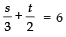(iii) 3x - y = 3, 9x − 3y = 9
(iv)
0.2x + 0.3y = 1.3, 0.4x + 0.5y = 2.3
(v) √2x + √3y = 0, √3x-√8y = 0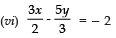,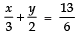Solution. (i) x + y = 14   ...(1)
x - y = 4    ...(2)
From the equation (1), we get
x = (14 - y)
Substituting this value of x in (2), we have:
(14 - y) - y = 4
⇒ 14 - 2y = 4
⇒- 2y = 4 - 14
⇒ - 2y = - 10 or y = 10/2
i.e., y = 5
Now, substituting y = 5 in (1), we have:
x + 5 = 14 ⇒ x = 14 - 5 ⇒ x = 9
Thus, x = 9
y = 5
(ii) s - t = 3   ...(1)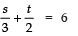...(2)
From (1), we have
s = (3 + t)    ...(3)
substituting this value of s in (2) we get: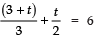= 6⇒ 2 (3 + t) + 3 (t) = 6 × 6 [multiplying throughout by 6]
⇒ 6 + 2t + 3t = 36
⇒ 5t = 36 - 6
⇒ 5t = 30 or t = 30/5 = 6
Substituting t = 6 in (3),
s = 3 + 6 = 9
Thus, s = 9
t = 6
(iii) 3x - y = 3   ...(1)
9x - 3y = 9   ...(2)
From the equation (1),
y = (3x - 3)
Substituting this value of y in (2),
9x - 3 (3x - 3) = 9
⇒ 9x - 9x + 9 = 9
⇒ 9 = 9
which is true,
∴ The equations (1) and (2) have unlimited solutions.
(iv) 0.2x + 0.3y = 1.3     ...(1)
0.4x + 0.5y = 2.3     ...(2)
From the equations (1), we get
0.3y = (1.3 - 0.2x)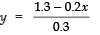..(3)
From (2) and (3), we have: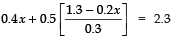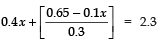⇒ 0.3 × 0.4x + 0.65 - 0.1x = 0.3 × 2.3
⇒ 0.12x + 0.65 - 0.1x = 0.69
⇒ 0.12x - 0.1x = 0.69 - 0.65
⇒ 0.02x = 0.04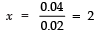From (iii),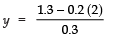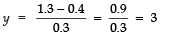Thus, x = 2 and y = 3
(v)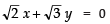...(1)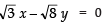...(2)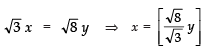...(3)
From (3) and (1), we have: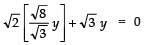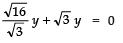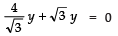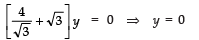Substituting y = 0 in (3), we have: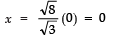Thus, x = 0 and y = 0.

(vi)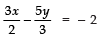...(1)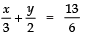...(2)
From (2), we have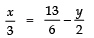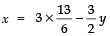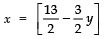...(3)
Substituting the value of x in (1),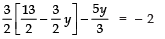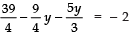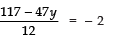⇒ 117 − 47y = − 24
⇒− 47y = − 24 − 117 = − 141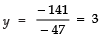Now, substituting y = 3 in (1), we have: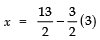Thus, x = 2 and y = 3.

Q.2. Solve 2x + 3y = 11 and 2x - 4y = - 24 and hence find the value of ‘m’ for which y = mx + 3.
Sol. We have 2x + 3y = 11 ...(1)
2x - 4y = - 24 ...(2)
From (1), we have:
2x = 11 - 3y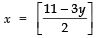...(3)
Substituting this value of x in (2), we have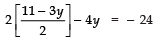⇒ 11 − 3y − 4y = − 24

⇒ 11 − 7y = − 24 ⇒− 7y = − 24 − 11 = − 35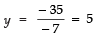Substituting y = 5 in (3), we get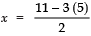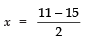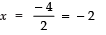∴ x = 2 and y = 5
Now, y = mx + 3
⇒ 5 = m (− 2) + 3
⇒ − 2 m = 5 − 3
⇒ − 2 m = 2
or m = -2/2 = -1
Thus, m = -1

Q.3. Form the pair of linear equations for the following problems and find their solution by substitution method.
(i) The difference between two numbers is 26 and one number is three times the other. Find them.
(ii) The larger of two supplementary angles exceeds the smaller by 18 degrees. Find them.
(iii) The coach of a cricket team buys 7 bats and 6 balls for ₹ 3800. Later, she buys 3 bats and 5 balls for ₹ 1750. Find the cost of each bat and each ball.
(iv) The taxi charges in a city consist of a fixed charge together with the charge for the distance covered. For a distance of 10 km, the charge paid is ₹ 105 and for a journey of 15 km, the charge paid is ₹ 155. What are the fixed charges and the charge per km? How much does a person have to pay for travelling a distance of 25 km?
(v) A fraction becomes 9/11, if 2 is added to both the numerator and the denominator. If, 3 is added to both the numerator and the denominator it becomes 5/6. Find the fraction.
(vi) Five years hence, the age of Jacob will be three times that of his son. Five years ago, Jacob’s age was seven times that of his son. What are their present ages?
Solution. (i) Let the two numbers be x and y such that x > y
∵ Difference of the numbers = 26
∴  x - y = 26    ...(1)
Again
One number = 3 [the other number]
⇒  x = 3y  ...(2)
Substituting x = 3y in (1), we get
3y - y = 26
⇒ 2y = 26 ∴ y = 26/2 = 13
Now, substituting y = 13 in (2), we have:
x = 3 (13)
⇒ x = 39
Thus, the required solution is:
x = 39  and  y = 13
(ii) Let the two angles be x and y such that x > y
∵ The larger angle exceeds the smaller by 18°
∴ x = y + 18°   ...(1)
Also, sum of two supplementary angles = 180°
∴ x + y = 180°   ...(2)
From (1), x = (18° + y)
Substituting this value of x in (2),
(18° + y) + y = 180°
⇒ 2y = 180° - 18° = 162°
⇒y =162º/2 = 81º
Substituting y = 81 in (1), we get
x = 18° + 81° = 99°
Thus x = 99°  and  y = 81°
(iii) Let the cost of a bat = ₹ x
And the cost of a ball = ₹ y
∵ [cost of 7 bats] + [cost of 6 balls] = ₹ 3800
⇒ 7x + 6y = 3800  ...(1)
Again
[cost of 3 bats] + [cost of 5 balls] = ₹ 1750
⇒ 3x + 5y = 1750   ..(2)
From (2), we have: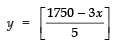Substiuting this value of y in (1), we have: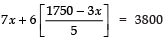⇒ 35x + 6 (1750 - 3x) = 5 × 3800
⇒ 35x + 10500 - 18x = 19000
⇒ 17x = 19000 - 10500
⇒ x = 8500/17 = 500
Substituting x = 500 in (3), we have: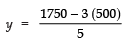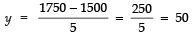y =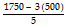⇒ y =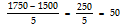Thus, x = 500  and  y = 50
⇒ Cost of a bat = ₹ 500
Cost of a ball = ₹ 50
(iv) Let fixed charges be ₹ x
And charges per km be ₹ y
∵ Charges for the journey of 10 km = ₹ 105
∴  x + 10y = 105    ...(1)
Again, charge for the journey of 15 km is ₹ 155.
∴ x + 15y = 155   ...(2)
From (1), x = (105 - 10y)   ...(3)
From (2) and (3),
(105 - 10y) + 15y = 155
⇒ 5y = 155 - 105 = 50
⇒ y = 50/5 = 10
Substituting y = 10 in (3), we get
x = 105 - 10 (10)
⇒x = 105 - 100 = 5
Thus, x = 5  and  y = 10
⇒ Fixed charges = ₹ 5
Charges per km = ₹ 10
Now, Charges for 25 km
= x + 25y
= 5 + 25 (10)
= 5 + 250 = 255
∴The charges for 25 km journey = ₹ 255
(v) Let the numerator = x
And the denominator = y
⇒ Fraction = x/y
Case I: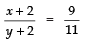⇒ 11 (x + 2) = 9 (y + 2)
11x + 22 = 9y + 18
⇒ 11x - 9y = 18 - 22
⇒11x - 9y + 4 = 0   ...(1)

Case II: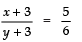⇒ 6 (x + 3) = 5 (y + 3)
⇒ 6x + 18 = 5y + 15
⇒ 6x - 5y + 18 - 15 = 0
⇒ 6x - 5y + 3 = 0   ...(2)
Now, from (2),
x =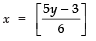...(3)
Substituting this value of x in (1), we get: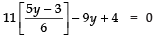⇒ 11 × 5y - 11 × 3 - 6 × 9y + 6 × 4 = 0
55y - 33 - 54y + 24 = 0
y - 9 = 0
y = 9
Now, substituting y = 9 in (3), we get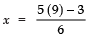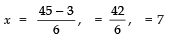∴ x = 7 and y = 9
⇒ Fraction = 7/9·
(vi) Let the present age of Jacob = x years.
And the present age of his son = y years.
∴ 5 years hence
Age of Jacob = (x + 5) years
Age of his son = (y + 5) years
Condition:
[Age of Jacob] = 3 [Age of his son]
∴ x + 5 = 3 (y + 5)
x + 5 = 3y + 15
x - 3y = 15 - 5
x - 3y - 10 = 0  ...(1)
5 years ago:
Age of Jacob = (x - 5) years
Age of his son = (y - 5) years
Condition:
[Age of Jacob] = 7 [Age of his son]
∴ (x - 5) = 7 (y - 5)
x - 5 = 7y - 35
x - 7y = - 35 + 5 = - 30
x - 7y + 30 = 0     ...(2)
From (1), x = [10 + 3y]   ...(3)
Substituting this value of x in (2),
(10 + 3y) - 7y + 30 = 0
⇒ 10 + 3y - 7y + 30 = 0
⇒ - 4y = - 40
⇒ y = -40/40 = 10
Now substituting y = 10 in (3), we get
x = 10 + 3 (10)
⇒ x = 10 + 30 = 40
Thus, x = 40  and  y = 10
⇒ Present age of Jacob = 40 years
⇒ Present age of his son = 10 years.

l Elimination Method
In elimination method, we eliminate one of the unknown quantities by using the following steps.
I. The given equations are multiplied by a suitable number so that the coefficients of one of the variables become numerically equal.
II. If the numerically equal coefficients are opposite in sign then add the new equations, otherwise, subtract them.
III. Solve the resulting linear equation to get the value of one of the variables.
IV. Substitute this value in any of the given equations and get the value of the other variable.

Offer running on EduRev: Apply code STAYHOME200 to get INR 200 off on our premium plan EduRev Infinity!

## Mathematics (Maths) Class 10

178 videos|268 docs|103 tests

,

,

,

,

,

,

,

,

,

,

,

,

,

,

,

,

,

,

,

,

,

;